### 1,1,2,3,5,8,13,21...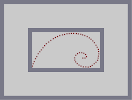Hover over the thumbnail for a full-size version.

Author protectedlord author:protectedlord cool-tastic curves golden n-art rated ratio 2009-04-08 2009-04-08 4 by 25 people. \$1,1,2,3,5,8,13,21...#protectedlord#none#00000000000000000000000000000000000000000000000000000000000000000000000000000000000000000000000000000000000000000000000000000000000000000000000011111111111000000000000100000000010000000000001000000000100000000000010000000001000000000000100000000010000000000001000000000100000000000010000000001000000000000100000000010000000000001000000000100000000000010000000001000000000000100000000010000000000001000000000100000000000010000000001000000000000100000000010000000000001000000000100000000000010000000001000000000000100000000010000000000001000000000100000000000011111111111000000000000000000000000000000000000000000000000000000000000000000000000000000000000000000000000000000000000000000000000000000000000000000000000|12^192,408!12^486,396!12^474,390!12^195,396!12^199,382!12^205,369!12^213,352!12^221,339!12^229,322!12^239,310!12^258,286!12^388,206!12^402,202!12^415,201!12^428,200!12^442,200!12^458,201!12^470,203!12^593,323!12^591,336!12^589,348!12^586,361!12^576,373!12^565,382!12^553,392!12^529,402!12^515,401!12^542,399!12^500,401!12^463,381!12^454,371!12^592,309!12^485,205!12^496,209!12^509,213!12^523,219!12^292,252!12^278,262!12^268,274!12^248,299!12^305,242!12^320,232!12^332,225!12^345,219!12^359,213!12^375,209!12^491,316!12^503,319!12^590,296!12^583,283!12^536,227!12^546,235!12^557,245!12^566,256!12^576,271!12^449,360!12^448,347!12^465,322!12^477,316!12^455,331!12^514,327!12^519,340!12^510,348!12^497,348# Kudos if you recognize it. Mathematically, this is a beautiful map. my first N-art... (getting the curve and proportions right was a challenge(check it your self) - this took a lot longer then it looks.)

## Other maps by this author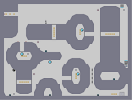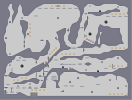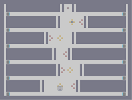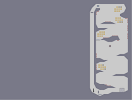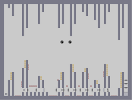Butane. Public Ally The Trickle Effect. Rockets (remeber those candys?) Lost? Bullet Drive

### Oh, nice.

Fibonacci spiral! I drew one of these during English class the other day.

### Hahaha

I'm surprised this map got an average of 3: The ignorant rated it 0 and the informed ignored the rating and just started exchanging mathematical proofs.

### Well,

technically there is a right and wrong way.
I always manage to screw it up though.

### Actually, It just really

depends on your way of rounding.
Yeah, I guess that is the easiest proof.

Sum(k=1..inf){10^-k} = 1/9, therefore 9*Sum(k=1..inf){10^k} = 1

Another one-step proof for .99999 = 1 is...
By the Geometric Series Theorum, since |1/10| < 1
9*Sum(k=0..inf){10^-k} = 9/(1-(1/10)) = 9/(9/10) = 1/(1/10) = 10
.: 9.9999... = 10
.: 0.9999... = 1

### MATHS IS NOT BROKEN!

@_Null
"If .3333... (or 1/3) + .6666... (or 2/3), then shouldn't .9999... = 1?

Or this (Try to follow these steps):
Given A=B
1. Multiply both sides by A: A*A=A*B
2. Subtract B^2 from both sides: A^2 - B^2=A*B-B^2
3. Factor: (A+B)*(A-B)=B(A-B)
4. Simplify: A+B=B
5. Substitute A for B: B+B=B
6. 2B=B
7. Divide both sides by B: 2=1

I BROKE MATH!!!! AAHHHH!!!!"

STEP 4 CANNOT BE DONE BECAUSE YOU COULD BE DIVIDING BY 0.
I saw this on a poster at school.

### The golden ratio!!

Cool. You should've used gold for the curve though.
No. It just chose not to be seen. It's a ninja. One of the ones that fell off the rating of this map... which I still haven't rated.

it did... crazy.

### @ exuberance

Yeah, well there are also 10 kinds of people in this world: those that understand binary and those that don’t. lol my cheese comment got deleted :(
WOO!! FLAME WAR!! *pulls out rocket launcher*

...pulls out from WHERE? Well my inventory. Duh.

SHUTDOWN!

### Skippy

your ignorant, why don't you look up what the golden spiral represents. It means more than your little mind can fathom I suppose.

### Again...

wt*... is this about? a spiral of mines man, all u, why are u still posting...

All this is bla bla blah over a ****en spiral of mines.

Mines and like wat, 45 comments? the thing

doesnt

represent

any

thing

U all are making a big fuss over something that wont even change anything.

U sound like nerds (why? because i got no idea what this map is for and what u guys are saying...)

### If your comment was for

me exuberance that was clearly a presumption in my argument but as you said a reacurring decimal is obviously convergent nobody would dissagree so I though it reasonable whereas many people do try to argue the case for 1 as seperate from 0.999... and therefor its isn't obvious to everyone see the topic in the forums for example.
gah! Forgot that NUMA kills brackets thinking it's BBcode <_<

Imagine every lim is lim{n->inf}, the SUM(n=1..inf) is SUM(n=1..inf){9*10^-n} and SUM(9*10^-n) is SUM(n=1..inf){9*10^-n}
Yes, the proof in the last post is incorrect. It does have the right conclusion, but when you say .99999... what you mean is SUM(n=1..inf). And although it is very obvious looking at it that this converges to a number (it should also be obvious that it converges to 1), you still need to actually prove that. Obviously it's not going to diverge to infinity, but you still need to prove it until you can subtract the 2 sums since you cannot subtract divergent series.

It is easy to prove that this series converges, however. Consider the 0.99999... series. (the 9.9999... is just 10 times that). The n+1st term is 9*10^(-n-1) and the nth term is 9*10^n. Now, use the ratio test to find lim(|(-n-1)/-n|) = lim(|(9*10^(-n-1))/(9*10^-n)|) = lim(|10^-1|) = lim(0.1) = 0.1. And since 0.1 < 1, therefore the series converges absolutely. You can now subtract the series and continue with your proof.

Therefore SUM(9*10^-n) = 1.

### So is it

the golden ratio spiral or the fibonacci spiral the difference is important.
you might find this map interesting http://nmaps.net/151888

As for _Null your logic is flawed
to get from steps 3 and 4 you devide by (A-B)
however if A=B then A-B=0
any argument which contains devision by 0 is nonsence and will lead to contradictions that isn't anyting new and you haven't broken maths you've just broken the rules of maths. If you break the rules of maths you can "prove" anything = anything you want.

Your first argument is better I think there is a topic on it in the forums I like this version of it
a=0.999...
10a=9.999...
9a=10a-a
=9.999...-0.999...
=9
a=1

### Well...

If .3333... (or 1/3) + .6666... (or 2/3), then shouldn't .9999... = 1?

Or this (Try to follow these steps):
Given A=B
1. Multiply both sides by A: A*A=A*B
2. Subtract B^2 from both sides: A^2 - B^2=A*B-B^2
3. Factor: (A+B)*(A-B)=B(A-B)
4. Simplify: A+B=B
5. Substitute A for B: B+B=B
6. 2B=B
7. Divide both sides by B: 2=1

I BROKE MATH!!!! AAHHHH!!!!

As for the spiral, it looks great. 4/5

### >.<

This map doubled my total ratings.

### Yea,

and lol check out my maps. (I like cheese to)
There is one type of person in this world:
Those who start counting from 0
and those who don't

### Oye!!

The map may not be spectacular, but you truly have to be intellectual to appreciate the fibonacci. So to all you haters, I pity the fool.

### Ouch.

And yes it does really matter - ideas like the golden ratio are important. It's part of a more humanistic view that not many people embrace anymore :(
(although I don't understand why it's getting this much attention)

### Wow, cause this really matters

What a waste of time, it's depressing that people would rate and comment on this compared to some of the other maps. I'm not sniping I'm just giving it a 0.

THE THREAD IS TOO NERDY!! QUICK, DILUTE IT WITH A CURVE-RELATED DOUBLE ENTENDRE OR A SPAMMY ADVERSITEMENT OR A SNIPER WAR OR AN IRRELEVANT COMMENT ABOUT CHEESE!

### ha this is simple

trying to find an equation on a three dementional graph and knowing the types of equations out there to figure it out, o and logarythims and inverses are also in the bunch of equation finding

### nobody's going to check your work

i think its stupid how something like this got so many rates.

still, kudos to you if you did go thru the trouble, i just dont think it would be worth it.

### Nerds UNITE!!!

Surprisingly my picture is an umlauted 'o'. Coincidence exuberance? I think not!!!
BLAST!! It got rid of the square brackets in my last post, so now it's a segmentation fault! A thousand curses upon you foolish NUMA!! I"LL BE BACK!!

(for the record maps->nerdiness sould be maps(163043)->nerdiness but with brackets instead of parentheses)
#include <stdio.h>
#include "NUMA.h"

int main() {
printf("Nerdiness: %d",maps->nerdiness);
return(0);
}

-------

\$ gcc -std=c99 -O -o nerdcheck nerdcheck.c NUMA.c

\$ ./nerdcheck
Nerdiness: 2147483647

### ...

WHAT THE **** IS SO COOL ABOUT A SPIRAL?

Seriously 30 comments over a spiral of mines, what does it represent anyway?

To hell, 30 comments. I don't see anything. NR...
When rounding to the nearest number for a number k+.5 for k an integer, there's 2 main ideas:

1) always round up
2) round to the even number

I propose a new strategy!
Do not round 2.5 to 2 or to 3. round it to both 2 AND 3! 2.5 = 2+ö where ö is simultaneously 0 AND 1. I dub this contradiction of a number Schrödinger's number! HOORAY!

Yes, I just created quantum numbers! That's even worse than imaginary numbers since they make no sense!

### My Statement still stands

He says almost perfect so 4.499999, would round up to 4.5.
Now 4.59 would round up to 5.

yay!

### Hmm...

On the subject of rounding I though that it was in practise to round up at 5s but in reality to be fair we should round up some of the time and down other times. This is because for example numbers ranging from 1 to 2.

10o does not round... nor does 2.0. They are already solid.
.1, .2, .3, .4 all round down. So 4 numbers round down. But 5 round up:
.5, .6, .7, .8 and .9. So really you should round up half the time and down the other half with 5s so that it becomes 4.5 numbers round up and 4.5 numbers round down... I think

Nice curve.

### Agreed.

Although I've really come to accept numa as a nerd community lmao